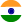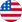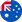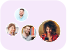24*7 support on WhatsAppChat NowProduct Edition:10th Edition
Author: Sheldon M. Ross
Book Name: Introduction to Probability Models
Subject Name: Maths

# Introduction to Probability Models 10th Edition Solutions

0 out of 5.0
34 reviews24 Students
have requested for homework help from this book

Introduction to Probability Models Tenth Edition provides an introduction to elementary probability theory and stochastic processes. There are two approaches to the study of probability theory.One is heuristic and nonrigorous and attempts to develop in students an intuitive feel for the subject that enables him or her to think probabilistically. The other approach attempts a rigorous development of probability by using the tools of measure theory. The first approach is employed in this text.The book begins by introducing basic concepts of probability theory such as the random variable conditional probability and conditional expectation. This is followed by discussions of stochastic processes including Markov chains and Poison processes. The remaining chapters cover queuing reliability theory Brownian motion and simulation. Many examples are worked out throughout the text along with exercises to be solved by students.This book will be particularly useful to those interested in learning how probability theory can be applied to the study of phenomena in fields such as engineering computer science management science the physical and social sciences and operations research. Ideally this text would be used in a one-year course in probability models or a one-semester course in introductory probability theory or a course in elementary stochastic processes.New to this Edition:65% new chapter material including coverage of finite capacity queues insurance risk models and Markov chainsContains compulsory material for new Exam 3 of the Society of Actuaries containing several sections in the new examsUpdated data and a list of commonly used notations and equations a robust ancillary package including a ISM SSM and test bankIncludes SPSS PASW Modeler and SAS JMP software packages which are widely used in the fieldHallmark features:Superior writing styleExcellent exercises and examples covering the wide breadth of coverage of probability topicsReal-world applications in engineering science business and economicsRead more

5
17
4
7
3
7
2
0
1
3

0

## Students who viewed this book also checked out